# Solving Absolute Value Equations AII 4 a The

• Slides: 44Solving Absolute Value Equations AII. 4 a – The student will solve, algebraically and graphically, absolute value equations and inequalities. Graphing calculators will be used for solving and for confirming the algebraic solution.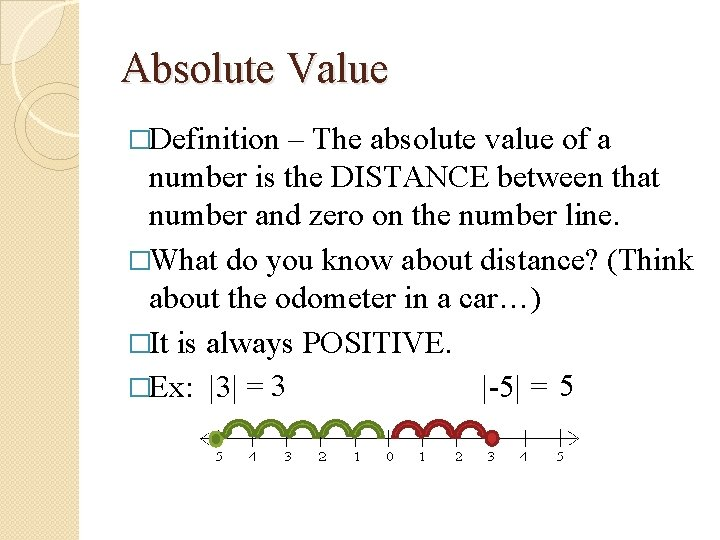Absolute Value �Definition – The absolute value of a number is the DISTANCE between that number and zero on the number line. �What do you know about distance? (Think about the odometer in a car…) �It is always POSITIVE. �Ex: |3| = 3 |-5| = 5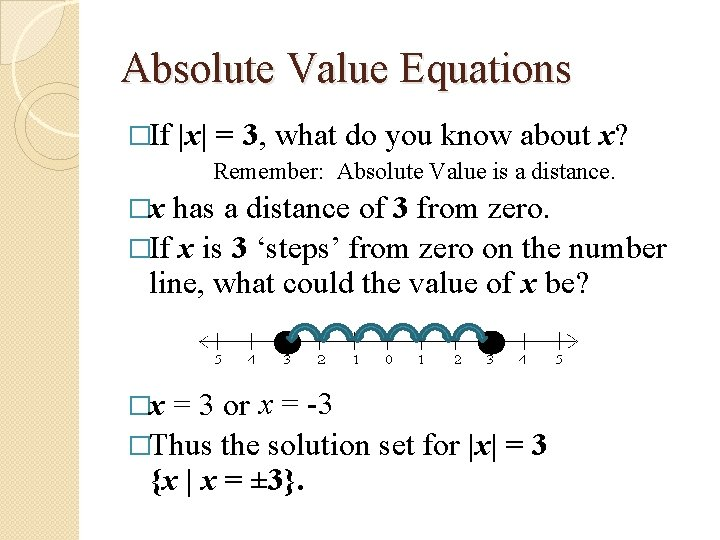Absolute Value Equations �If |x| = 3, what do you know about x? Remember: Absolute Value is a distance. �x has a distance of 3 from zero. �If x is 3 ‘steps’ from zero on the number line, what could the value of x be? = 3 or x = -3 �Thus the solution set for |x| = 3 {x | x = ± 3}. �x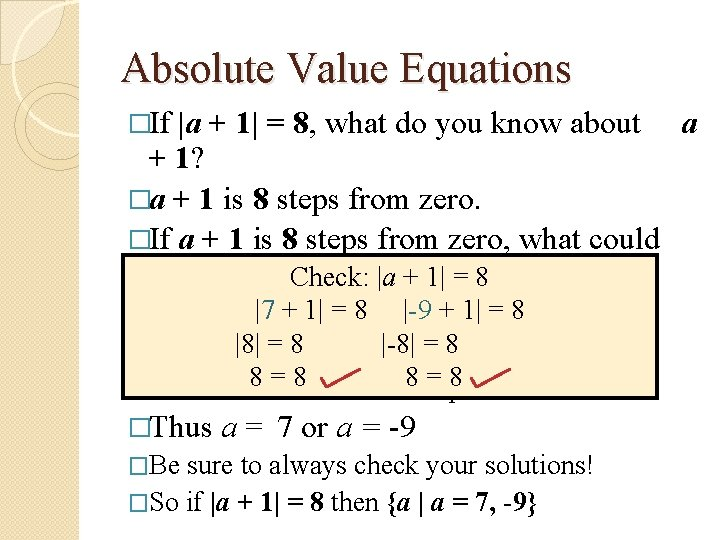Absolute Value Equations �If |a + 1| = 8, what do you know about a + 1? �a + 1 is 8 steps from zero. �If a + 1 is 8 steps from zero, what could the value of. Check: a + 1 |abe? + 1| = 8 |7 + 1| = 8 |-9 + 1| = 8 �a + 1 = ± 8 �a + 1 =|8|8 = 8 or |-8| = a 8+ 1 = -8 8 =Solve 8 these two 8 =equations… 8 �Thus �Be a = 7 or a = -9 sure to always check your solutions! �So if |a + 1| = 8 then {a | a = 7, -9}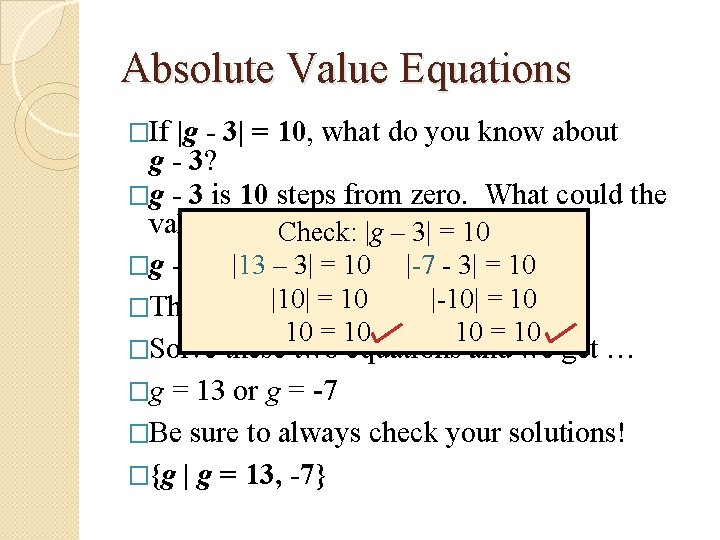Absolute Value Equations �If |g - 3| = 10, what do you know about g - 3? �g - 3 is 10 steps from zero. What could the value of g –Check: 3 be? |g – 3| = 10 �g - 3 = |13 ± 10– 3| = 10 |-7 - 3| = 10 �Thus g - 3|10| = 10 or g - 3|-10| = -10 10 = 10 �Solve these two equations and we get … �g = 13 or g = -7 �Be sure to always check your solutions! �{g | g = 13, -7}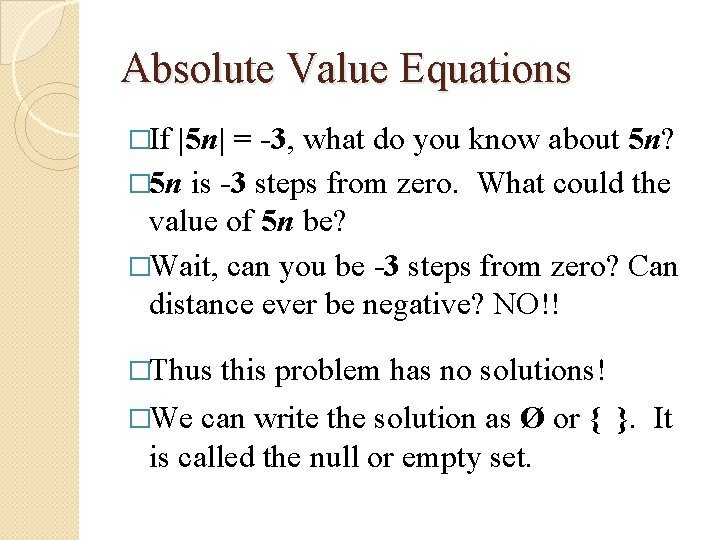Absolute Value Equations �If |5 n| = -3, what do you know about 5 n? � 5 n is -3 steps from zero. What could the value of 5 n be? �Wait, can you be -3 steps from zero? Can distance ever be negative? NO!! �Thus �We this problem has no solutions! can write the solution as Ø or { }. It is called the null or empty set.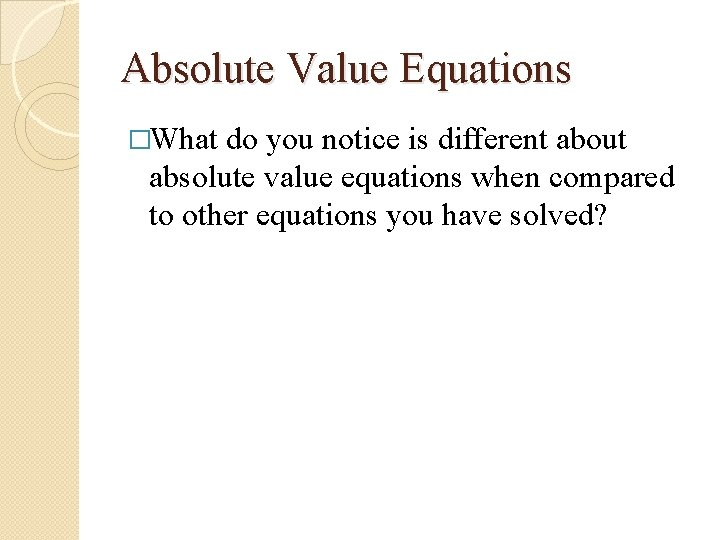Absolute Value Equations �What do you notice is different about absolute value equations when compared to other equations you have solved?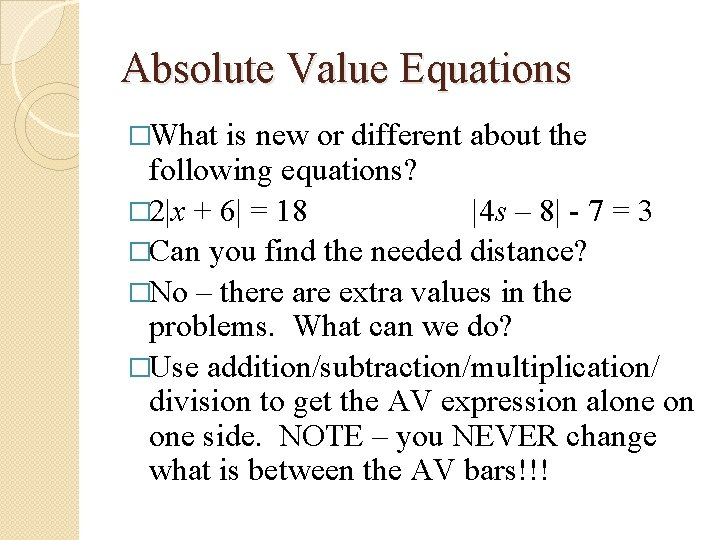Absolute Value Equations �What is new or different about the following equations? � 2|x + 6| = 18 |4 s – 8| - 7 = 3 �Can you find the needed distance? �No – there are extra values in the problems. What can we do? �Use addition/subtraction/multiplication/ division to get the AV expression alone on one side. NOTE – you NEVER change what is between the AV bars!!!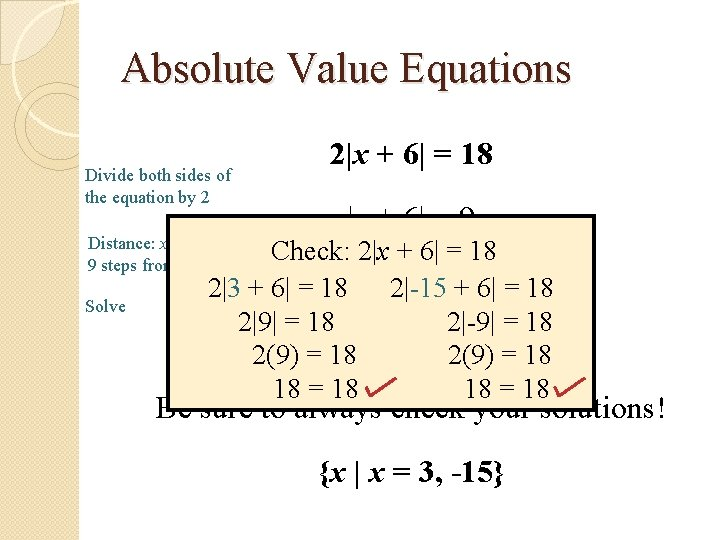Absolute Value Equations Divide both sides of the equation by 2 Distance: x + 6 is 9 steps from 0 Solve 2|x + 6| = 18 |x + 6| = 9 Check: 2|x + 6| = 18 = 9 or -9 2|3 +x 6|+=618 2|-15 x++6|6==18 2|9| = 18 2|-9| = 18 2(9) = 18 x = 3, -15 2(9) = 18 18 = 18 Be sure to always check your solutions! {x | x = 3, -15}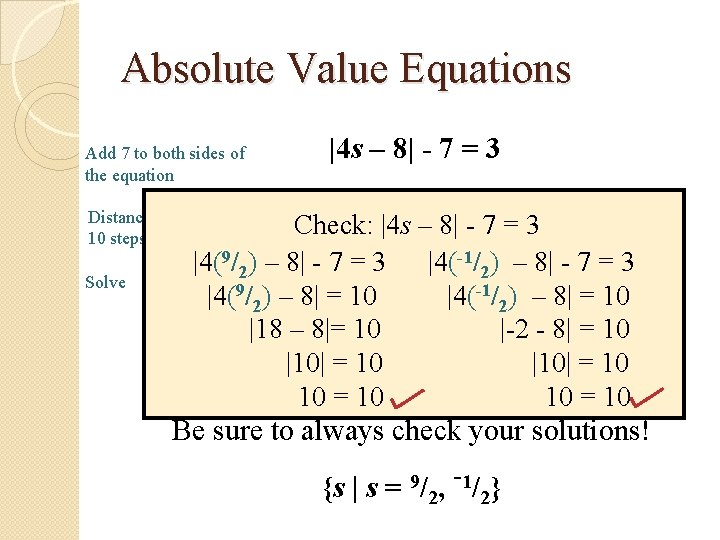Absolute Value Equations Add 7 to both sides of the equation Distance: 4 s – 8 is 10 steps from 0 Solve |4 s – 8| - 7 = 3 |4 s – 8| = 10 Check: |4 s – 8| - 7 = 3 -1/–) 8–=8|-10 – 8 = 10 or 4 s |4(9/4 s ) – 8| 7 = 3 |4( -7=3 2 2 |4(9/2) – 8| = 10 |4(-1/2) – 8| = 10 = 18 -2 - 8| = 10 |18 – 4 s 8|= 10 or 4 s =|-2 |10| = s 10 |10| = 10 = 9/2, -1/2 10 = 10 Be sure to always check your solutions! {s | s = 9/2, -1/2}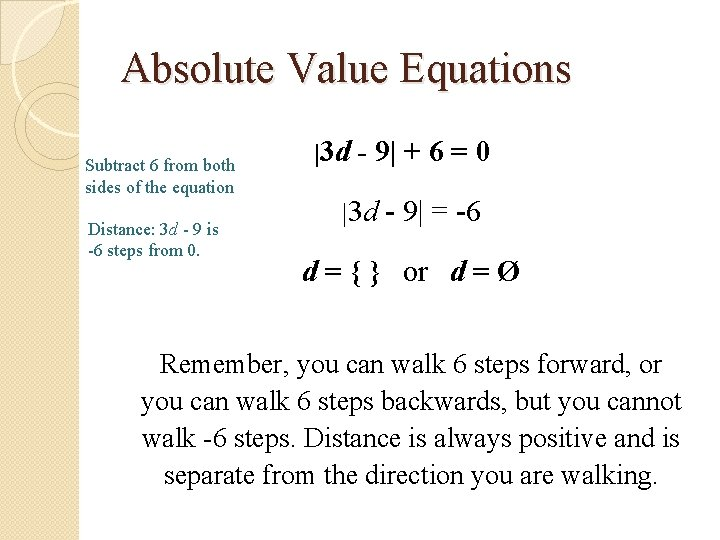Absolute Value Equations Subtract 6 from both sides of the equation Distance: 3 d - 9 is -6 steps from 0. |3 d - 9| + 6 = 0 |3 d - 9| = -6 d = { } or d = Ø Remember, you can walk 6 steps forward, or you can walk 6 steps backwards, but you cannot walk -6 steps. Distance is always positive and is separate from the direction you are walking.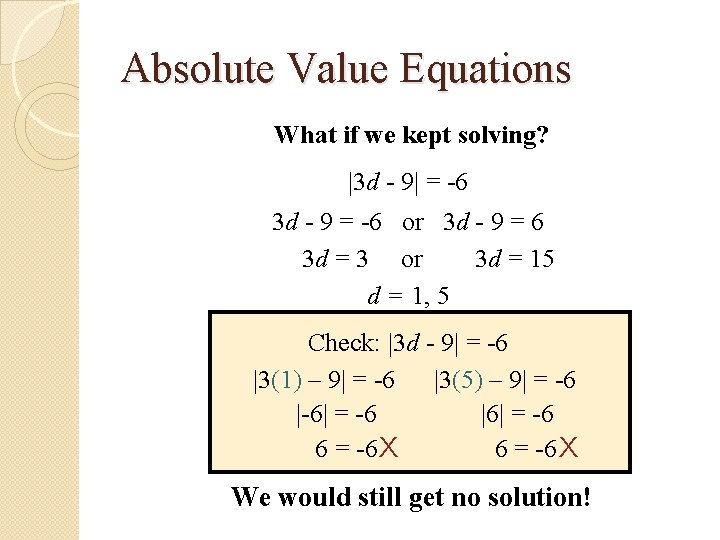Absolute Value Equations What if we kept solving? |3 d - 9| = -6 3 d - 9 = -6 or 3 d - 9 = 6 3 d = 3 or 3 d = 15 d = 1, 5 Check: |3 d - 9| = -6 |3(1) – 9| = -6 |3(5) – 9| = -6 |-6| = -6 |6| = -6 6 = -6 X We would still get no solution!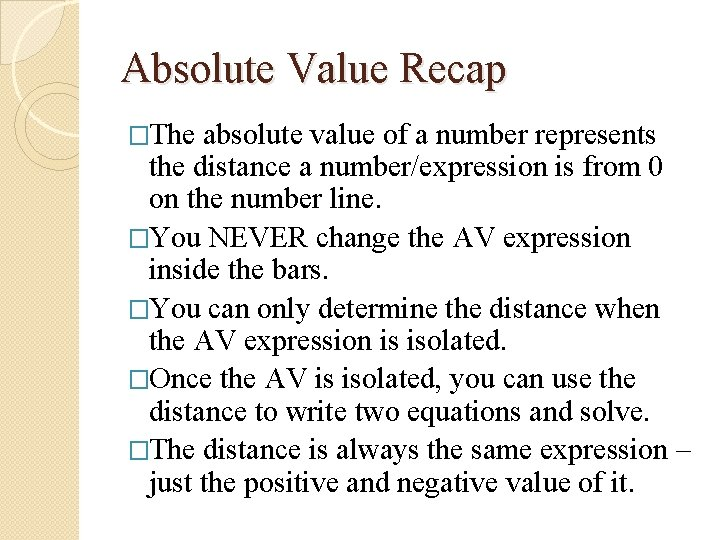Absolute Value Recap �The absolute value of a number represents the distance a number/expression is from 0 on the number line. �You NEVER change the AV expression inside the bars. �You can only determine the distance when the AV expression is isolated. �Once the AV is isolated, you can use the distance to write two equations and solve. �The distance is always the same expression – just the positive and negative value of it.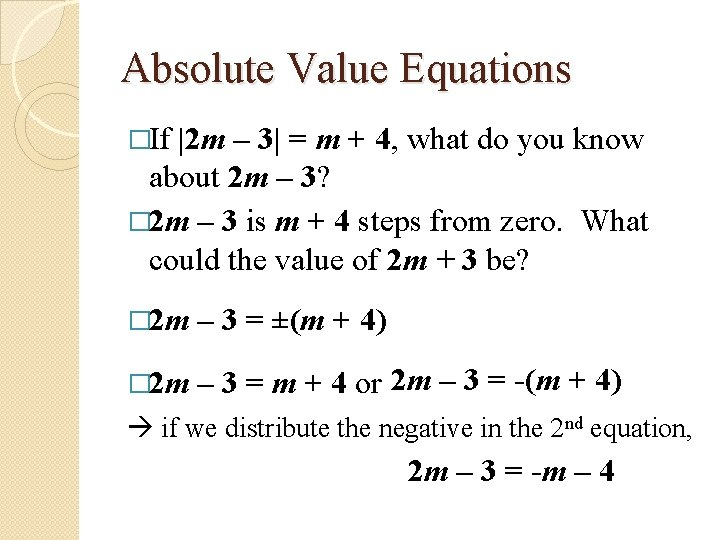Absolute Value Equations �If |2 m – 3| = m + 4, what do you know about 2 m – 3? � 2 m – 3 is m + 4 steps from zero. What could the value of 2 m + 3 be? � 2 m – 3 = ±(m + 4) � 2 m – 3 = m + 4 or 2 m – 3 = -(m + 4) if we distribute the negative in the 2 nd equation, 2 m – 3 = -m – 4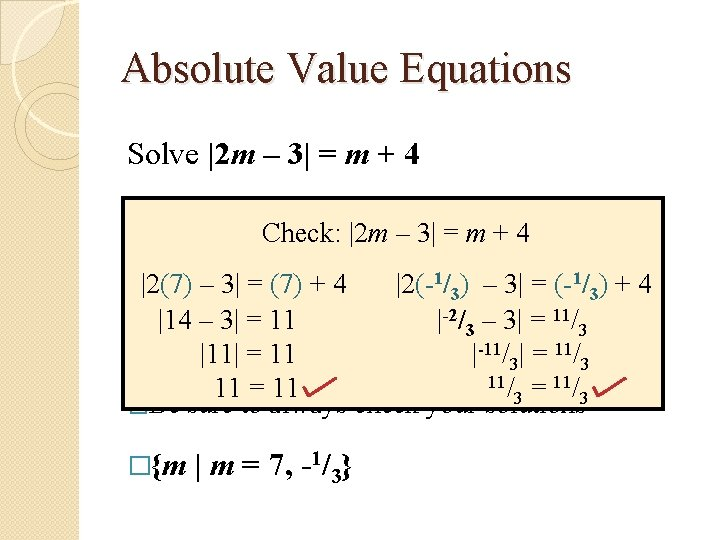Absolute Value Equations Solve |2 m – 3| = m + 4 2 m – 3 =Check: m + 4|2 m – 3| =2 m – 3 = -m – 4 m+4 7 +4 |2(7) – m 3| = (7) |2(-1/3) – 3 m 3| = = (-1 -1 /3) + 4 |14 – 3| = 11 |-2/3 – 3| = 11/3 �m = |11| 7, -=1/311 |-11/3| = 11/3 11/ = 11/ 11 = 11 3 3 �Be sure to always check your solutions �{m | m = 7, -1/3}Absolute Value Equations Distance: 8 + 5 a is 14 - a steps from 0 |8 + 5 a| = 14 - a 8 + 5 a = ±(14 – a) + 5 a|8=+14 8 + 5 a =Check: 14 – a |8 or 5 a-=a-(14 + a) Solve |8 + 5(1)| = 14 - 1 |8 + 5(-5. 5)| = 14 - (-5. 5) 8 + 5 a = 14 – a or 8 + 5 a = -14 + a |13| = 13 |-19. 5| = 19. 5 13 = 13 -11/ )= 19. 5 a = 1, -5. 5 (or 19. 5 2 Be sure to always check your solutions! {a | a = 1, -11/2}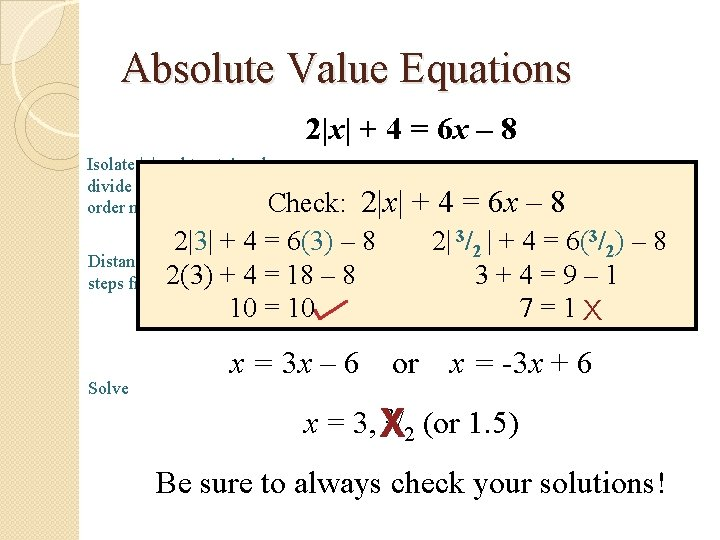Absolute Value Equations 2|x| + 4 = 6 x – 8 Isolate |x|: subtract 4 and divide by 2 (does this Check: order matter? ) 2|x| = 6 x – 12 2|x| + 4 = 6 x – 8 2|3| + 4 = 6(3) |x| – 8 = 3 x 2|– 3/62 | + 4 = 6(3/2) – 8 Distance: x is 3 x - 6 steps from 2(3) 0 Solve + 4 = 18 – 8 3+4=9– 1 10 = 10 x = ±(3 x – 6) 7 = 1 X x = 3 x – 6 or x = -3 x + 6 3/ (or 1. 5) x = 3, X 2 Be sure to always check your solutions!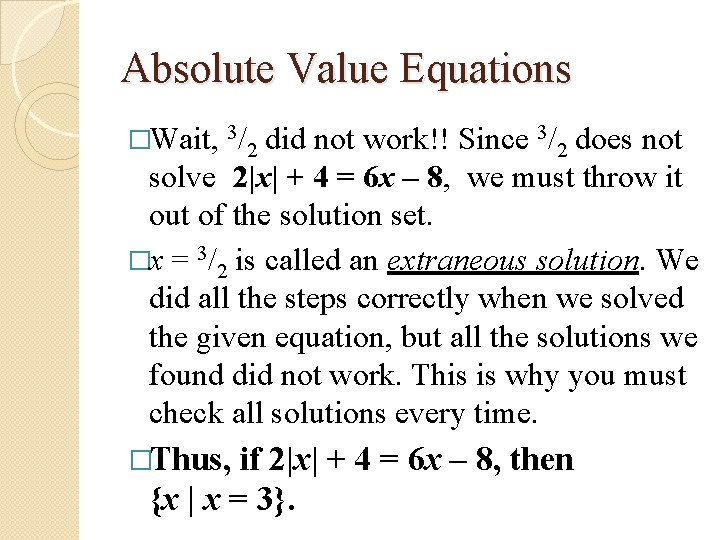Absolute Value Equations �Wait, 3/2 did not work!! Since 3/2 does not solve 2|x| + 4 = 6 x – 8, we must throw it out of the solution set. �x = 3/2 is called an extraneous solution. We did all the steps correctly when we solved the given equation, but all the solutions we found did not work. This is why you must check all solutions every time. �Thus, if 2|x| + 4 = 6 x – 8, then {x | x = 3}.Absolute Value Equations Distance: 3 x – 1 is 1 + 3 x steps from 0 Solve |3 x – 1| = 1 + 3 x 3 x – 1 = 1 + 3 x or 0=2 or Ø or 3 x – 1 = -1 – 3 x 6 x = 0 x=0 Be sure to always check your solutions! Check: |3 x – 1| = 1 + 3 x |3(0) – 1| = 1 + 3(0) |-1| = 1 1=1 {x | x = 0}How far … and from where? In carpentry, a stud is a vertical beam used to create support in a wall. Typically studs are positioned 2 feet apart. If there is a stud 8 feet from the intersecting wall, what are the positions of the studs on either side of the pictured stud (with respect to the intersecting wall)? 6 ft. and 10 ft. 8 ft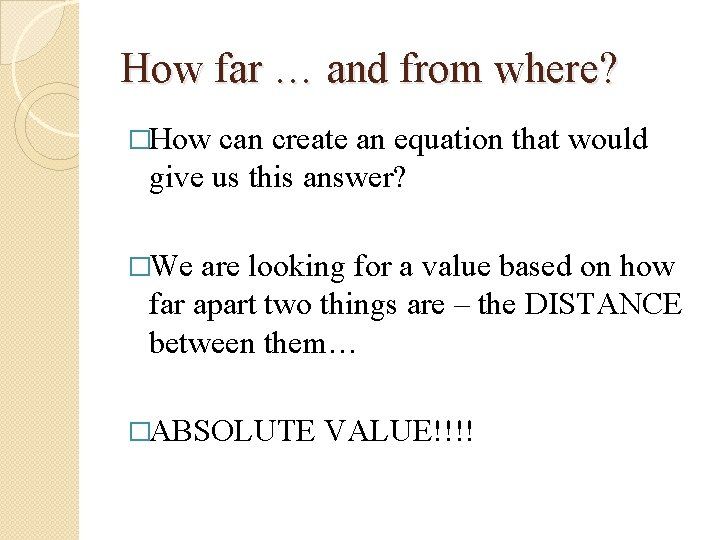How far … and from where? �How can create an equation that would give us this answer? �We are looking for a value based on how far apart two things are – the DISTANCE between them… �ABSOLUTE VALUE!!!!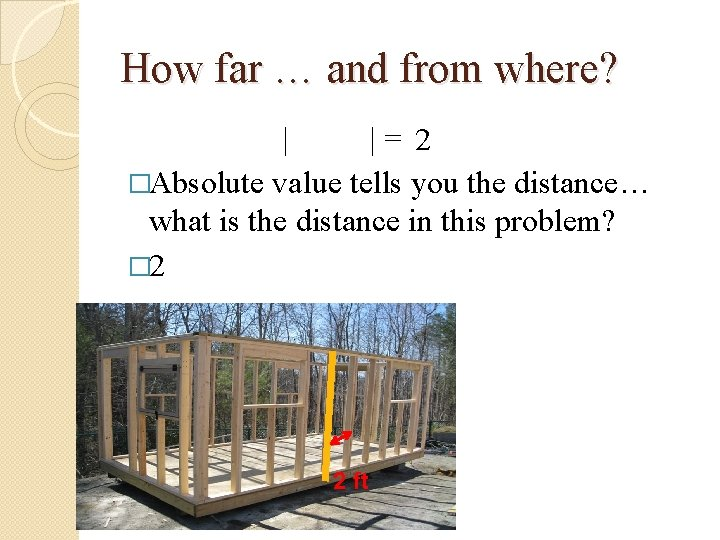How far … and from where? | |= 2 �Absolute value tells you the distance… what is the distance in this problem? � 2 2 ft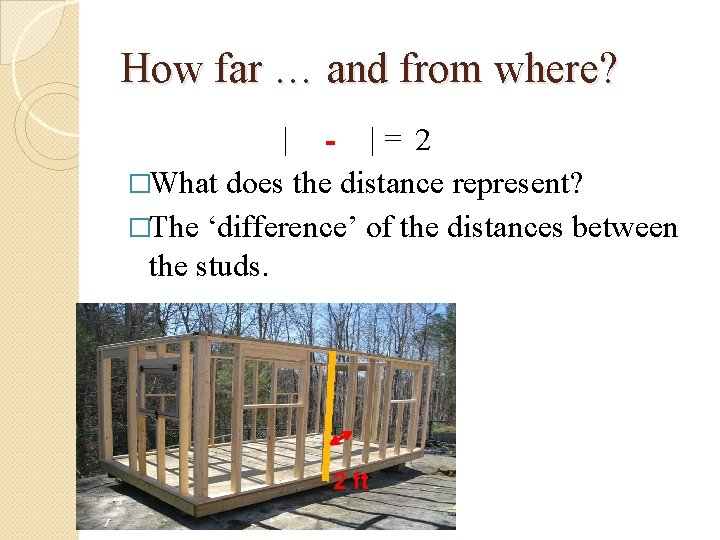How far … and from where? | - |= 2 �What does the distance represent? �The ‘difference’ of the distances between the studs. 2 ft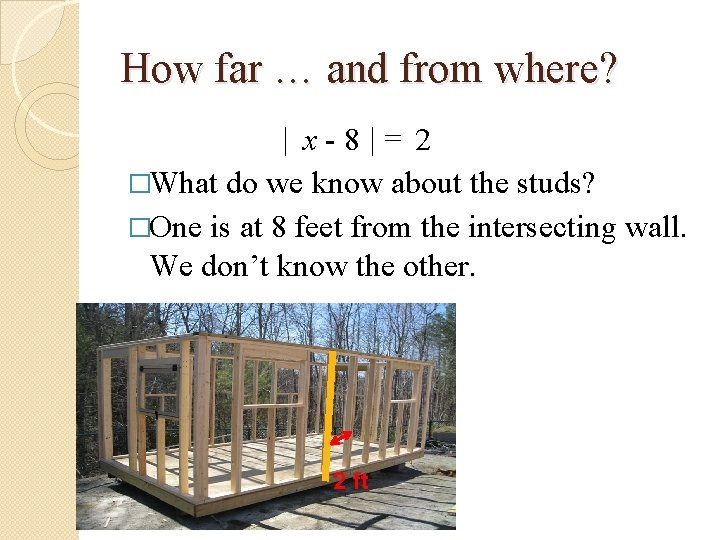How far … and from where? | x-8|= 2 �What do we know about the studs? �One is at 8 feet from the intersecting wall. We don’t know the other. 2 ft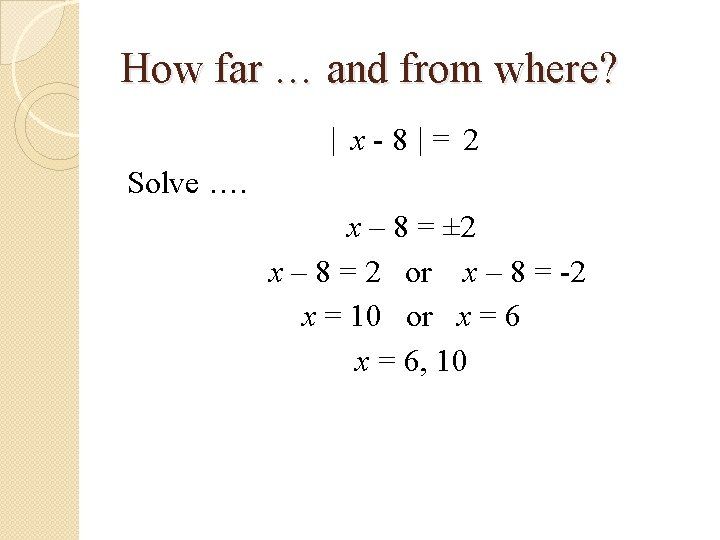How far … and from where? | x-8|= 2 Solve …. x – 8 = ± 2 x – 8 = 2 or x – 8 = -2 x = 10 or x = 6, 10How far … and from where? | x-8|= 2 We can generalize this to be: |unknown – given value| = distance |x – 8| = 2 Our solutions for x are the values 2 feet from 8.How far … and from where? |unknown – given value| = distance �What if we focused on a stud 5 feet from the intersecting wall? What would be the positions of the studs beside it? � 3 feet and 7 feet �Create an absolute value equation that would allow us to solve for these values. �|x - 5| = 2 �The solutions for x are the values 2 feet from 5.How far … and from where? �Looking at our previous problems again… �The solution set for |g - 3| = 10 is {g | g = 13, -7}. �Fill in the blanks … The values of g are ____ 10 steps from ____. 3 �Let’s prove this with the graph. 10 3 10How far … and from where? �The solution set for |a + 1| = 8 is {a | a = 7, -9}. �The values of a are ____ 8 steps from ____. -1 8 �The -1 8 solution set for |x + 6| = 9 (from 2|x + 6| = 18) is {x | x = 3, -15}. �The values of x are ____ 9 steps from ____. -6 9 9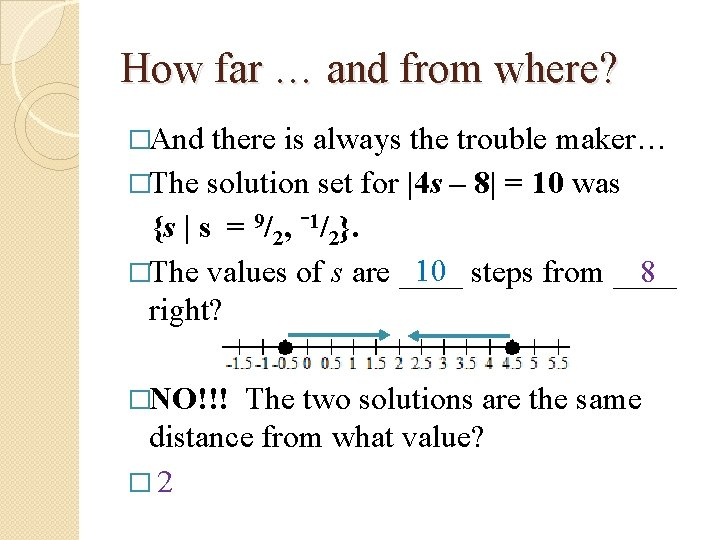How far … and from where? �And there is always the trouble maker… �The solution set for |4 s – 8| = 10 was {s | s = 9/2, -1/2}. 10 steps from ____ �The values of s are ____ 8 right? �NO!!! The two solutions are the same distance from what value? � 2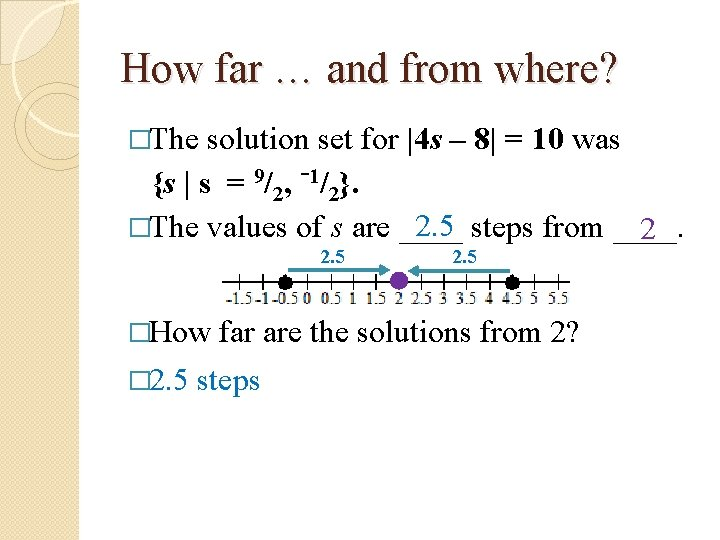How far … and from where? �The solution set for |4 s – 8| = 10 was 9 {s | s = /2, 1/2}. 2. 5 steps from ____. �The values of s are ____ 2 2. 5 �How � 2. 5 far are the solutions from 2? steps|unknown – given value| = distance How far … and from where? �The solution set for |4 s – 8| = 10 was 9 {s | s = /2, 1/2}. 2. 5 steps from ____. �The values of s are ____ 2 �Why is this equation different? �In the other equations, there was not a coefficient with the unknown variable. �We need to keep the coefficient with the unknown value.|unknown – given value| = distance How far … and from where? �The solution set for |4 s – 8| = 10 was {s | s = 1/2, 9/2}. �The values of 4 s are ____ 10 steps from ____. 8 �The values that are 10 steps from 8 are -2 and 18. �So 4 s = -2 and 4 s = 18. �Thus s = -½ and s = 18/4 = 9/2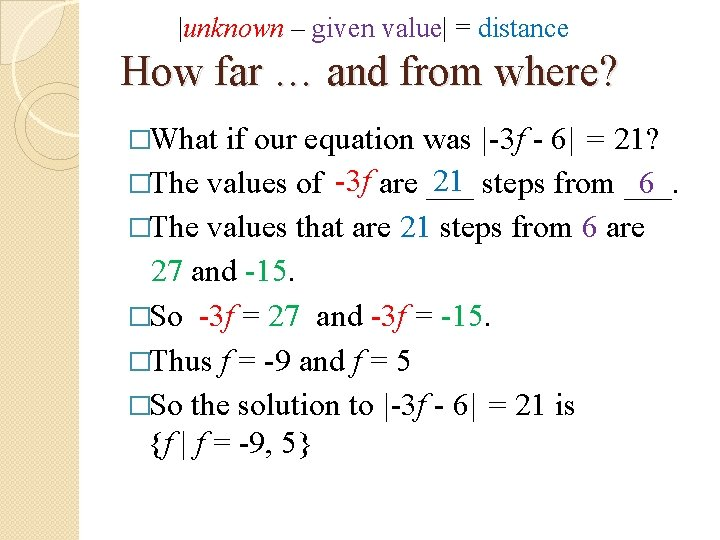|unknown – given value| = distance How far … and from where? �What if our equation was |-3 f - 6| = 21? 21 steps from ___. 6 �The values of -3 f are ___ �The values that are 21 steps from 6 are 27 and -15. �So -3 f = 27 and -3 f = -15. �Thus f = -9 and f = 5 �So the solution to |-3 f - 6| = 21 is {f | f = -9, 5}How far … and from where? �Going back to our very first example |x| = 3, the solutions are ____ 3 steps from ____. 0 �How does this fit into our generalized equation? |unknown – given value| = distance �The given value is 0 but we don’t need to write the equation as |x – 0| = 3.Examples Use the generalized form of an absolute value equation to solve each problem. �|3 m – 15| = 12 12 steps from ___. 15 �The values of 3 m are ___ 15 ± 12 = ? 3 m = 27 and 3 m = 3 m = 9 and m = 1 �Thus the solution set for |3 m – 15| = 12 is {m | m = 1, 9}.Examples �Solve: Use the generalized form of an absolute value equation to solve each problem. 2|5 h + 10| - 7 = 1 �Can we tell the distance here? �No, we must isolate the absolute value. �|5 h + 10| = 4 4 steps from ___. -10 �The values of 5 h are ___ �-10 ± 4 = -6, -14 � 5 h = -6 and 5 h = -14 �{h | h = -1. 2, -2. 8}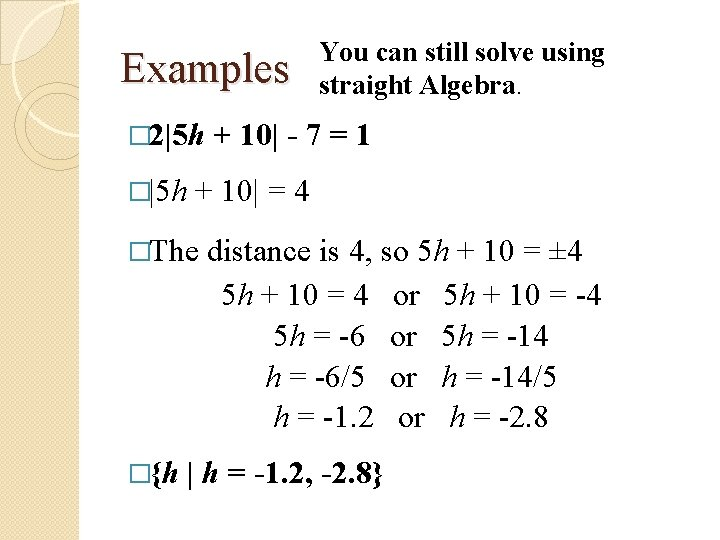Examples � 2|5 h �|5 h + 10| - 7 = 1 + 10| = 4 �The �{h You can still solve using straight Algebra. distance is 4, so 5 h + 10 = ± 4 5 h + 10 = 4 or 5 h + 10 = -4 5 h = -6 or 5 h = -14 h = -6/5 or h = -14/5 h = -1. 2 or h = -2. 8 | h = -1. 2, -2. 8}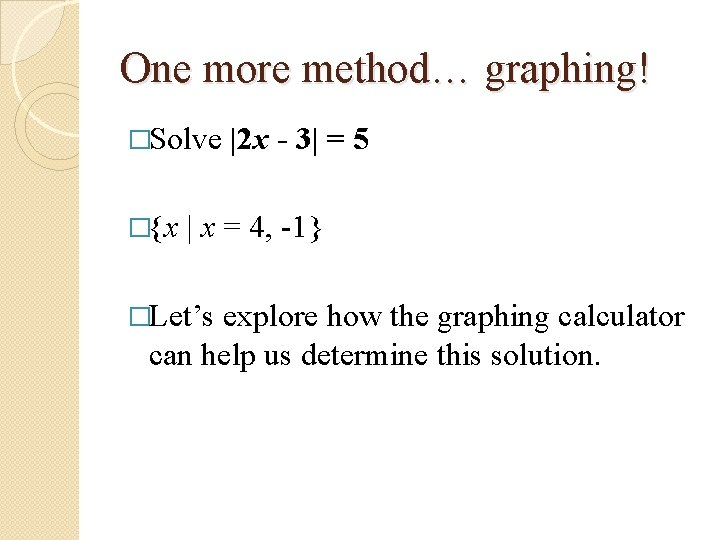One more method… graphing! �Solve �{x |2 x - 3| = 5 | x = 4, -1} �Let’s explore how the graphing calculator can help us determine this solution.Solve by Graphing �Graph y = |2 x - 3| and y = 5 using your graphing calculator. (See the next slides for directions. ) � My window: x-min: -4, x-max: 6; y-min: -3, y-max 7; all scales =1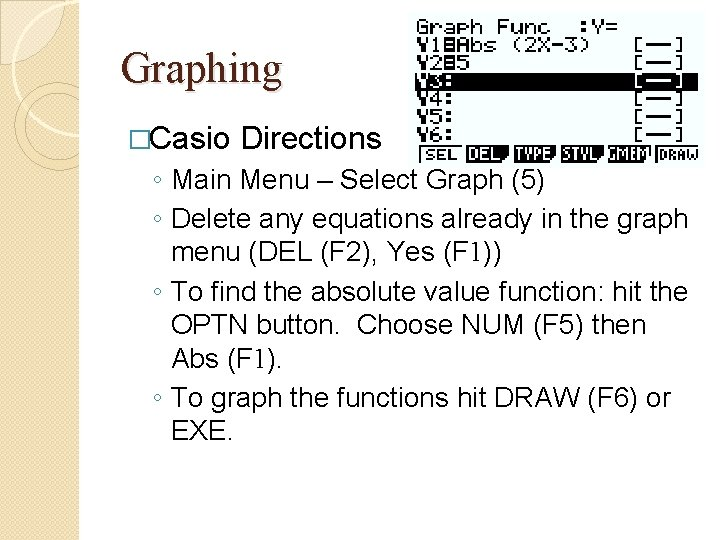Graphing �Casio Directions ◦ Main Menu – Select Graph (5) ◦ Delete any equations already in the graph menu (DEL (F 2), Yes (F 1)) ◦ To find the absolute value function: hit the OPTN button. Choose NUM (F 5) then Abs (F 1). ◦ To graph the functions hit DRAW (F 6) or EXE.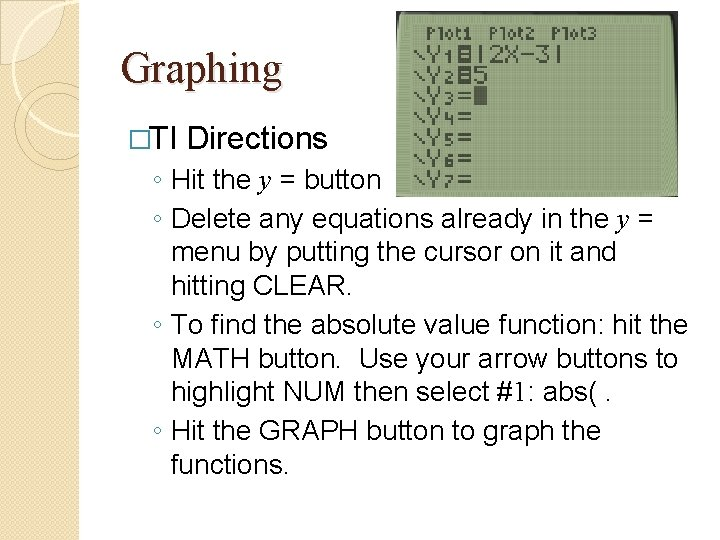Graphing �TI Directions ◦ Hit the y = button ◦ Delete any equations already in the y = menu by putting the cursor on it and hitting CLEAR. ◦ To find the absolute value function: hit the MATH button. Use your arrow buttons to highlight NUM then select #1: abs(. ◦ Hit the GRAPH button to graph the functions.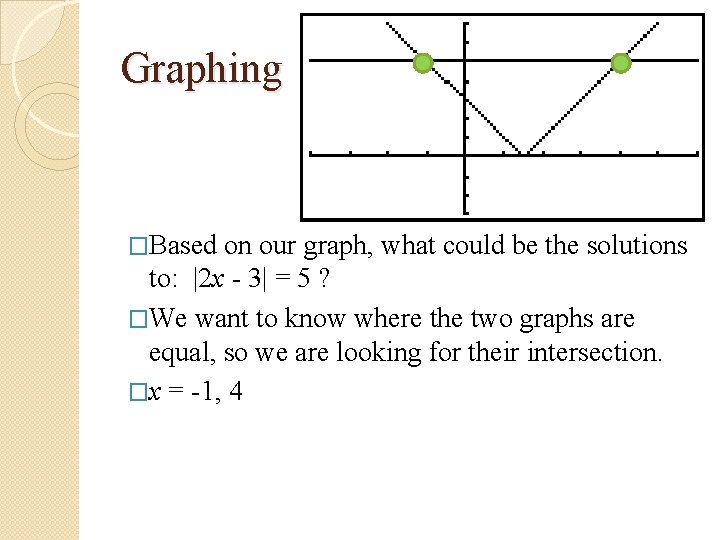Graphing �Based on our graph, what could be the solutions to: |2 x - 3| = 5 ? �We want to know where the two graphs are equal, so we are looking for their intersection. �x = -1, 4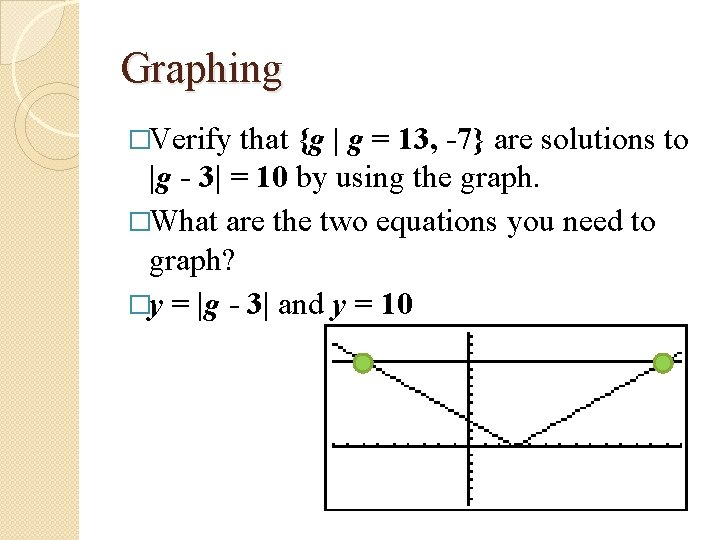Graphing �Verify that {g | g = 13, -7} are solutions to |g - 3| = 10 by using the graph. �What are the two equations you need to graph? �y = |g - 3| and y = 10